APPLIED INDUSTRIAL CONTROL SOLUTIONS LLCAPPLIED INDUSTRIAL CONTROL SOLUTIONS ApICS LLC. Digital Filter Design Writing Difference Equations For Digital Filters Brian T. Boulter © ApICS ® LLC 2000 ABSTRACT Difference equations are presented for 1st, 2nd, 3rd, and 4th order low pass and high pass filters, and 2nd, 4th and 6th order band-pass, band-stop and notch filters along with a resonance compensation (RES_COMP) filter. The low pass, high pass, bandpass and bandstop difference equations are obtained from the normalized butterworth continuous time filter descriptions given below. 1st. order normalized Butterworth low pass filter: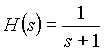(1.0a) 2nd. order normalized Butterworth low pass filter: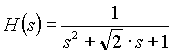(1.0b) 3rd. order normalized Butterworth low pass filter: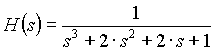(1.0c) 4th. order normalized Butterworth low pass filter: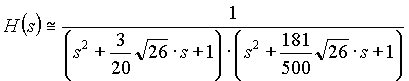(1.0d) 2nd. order normalized Notch filter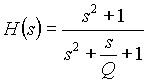1.1a) 4th order normalized Notch filter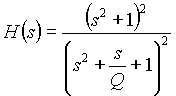(1.1b) 6th. order normalized Notch filter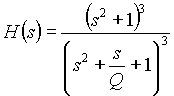(1.1c) Normalized Res_Comp filter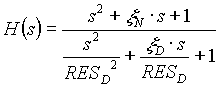(1.1d) The above low pass filter (not the notch or RES_COMP filters) equations are mapped to the appropriate filter type using the following mappings. To map (1.0x) to a lowpass filter use: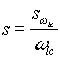;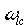= desired low-pass 3 [d.b.] cutt-off frequency. (1.2) To map (1.0x) to a highpass filter use: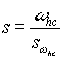;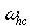= desired high-pass 3 [d.b.] pass frequency. (1.3) To map (1.0x) to a bandpass filter use: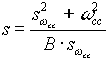(1.4) where: B = A number that controls the bandpass depth/width (equivelant to 1/Q).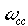= The desired continuous time center frequency. To Map (1.0x) to a band-stop filter use: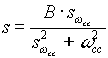(1.5) where: B = A number that controls the notch depth/width (equivelant to 1/Q).= The desired continuous time center frequency. For example if we want to obtain a fourth order band-stop filter we use equation (1.0d) and use the mapping of equation (1.5) after some algebra we obtain the following continuous filter description: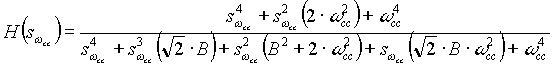To create a digital filter we normalize the above filter by setting= 1, and map to the z-domain using the bi-linear transformation: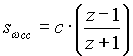(1.6) The c co-efficient in the above equation is used to accomplish frequency warping. That is, to compensate for an inherent inaccuracy in the bi-linear transformation method that is a function of frequency and sample rate. (see any D.S.P. text for a more complete explanation) Once the above mapping is performed by substituting (1.6) into the continuous time s-domain filter equation we obtain a z-domain transfer function of the form: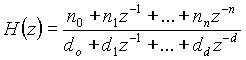(1.7) Where, for any filters obtained using the normalized low-pass butterworth filter as the basis for the digital filter, n = d = order of the filter. For low-pass to low-pass and low-pass to high-pass mapping the order of the digital filter is the same as the order of the starting normalized butterworth filter. For low-pass to band-pass and low-pass to band-stop mapping the order of the digital filter is twice the order of the starting normalized butterwoth low-pass filter. Let: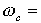the normalized notch center frequency. (i.e.1)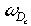= the desired notch center frequency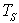= Sample time in sec. The warping equation is given as: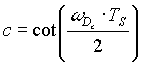(1.8a) The RES_COMP filter which uses the same mapping as above, requires that the denominator resonant frequency be post-warped with (1.8b) below.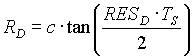(1.8b) After some algebra we obtain the following co-efficients in (1.7) for low-pass, high-pass, band-pass and band-stop filters for the indicated order of the filter. Low-Pass Filter n=1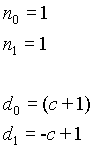n=2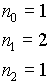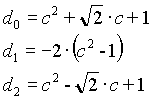n=3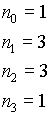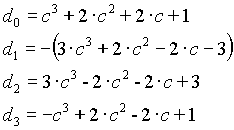n=4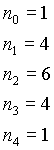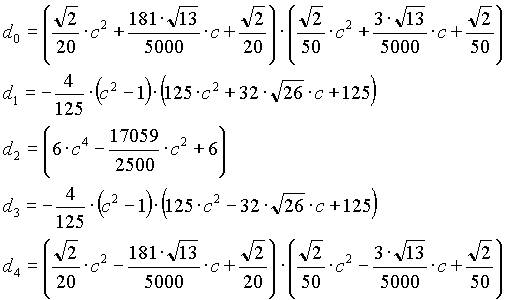High-Pass Filter n=1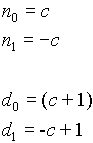n=2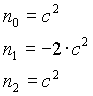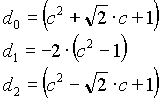n=3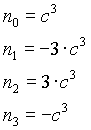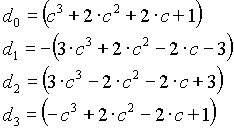n=4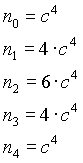Band-pass filter n=2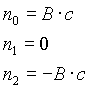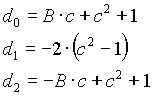n=4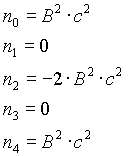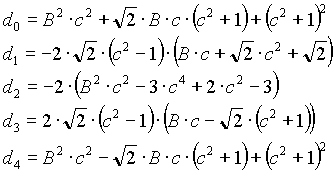n=6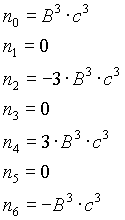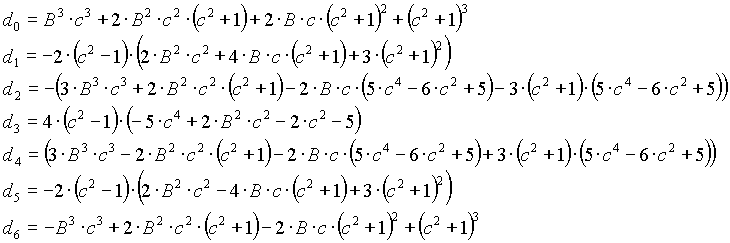Note: 1) B in the above equations is equavelant to 1/Q; where Q is the depth factor in notch filter designs. Band-stop filter n=2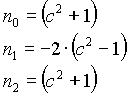n=4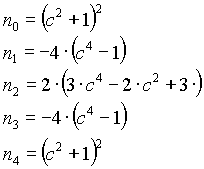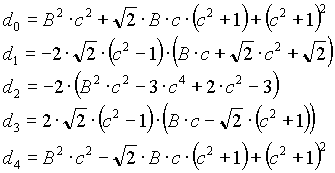n=6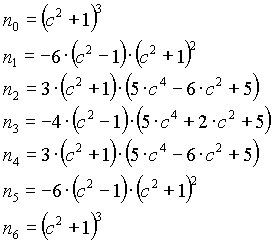Note: 1) B in the above equations is equavelant to 1/Q; where Q is the depth factor in notch filter designs. Notch filter n=2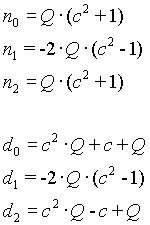n=4 Proprietary n=6 Proprietary RES_COMP filter Proprietary Notes: 1)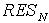is the zero compensation frequency with damping equal to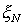/2. 2)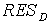is the pole compensation frequency with damping equal to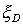/2. CREATING THE DIFFERENCE EQUATIONS: From the z-domain transfer function we obtain the difference equation as follows: Know from (1.7)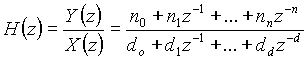(1.9) Therefore (1.9) becomes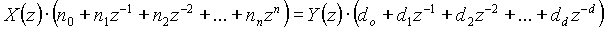In difference equation form: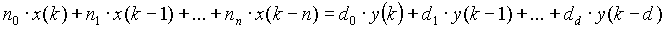or in terms of the latest output: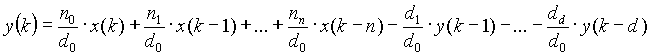(1.10) Where for each filter described above the appropriate co-efficients are used in (1.10).